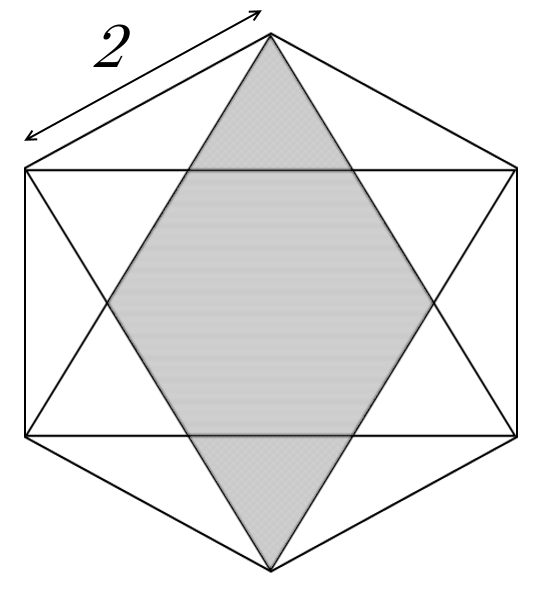# Horrible Hexagons 2

Geometry Level 3The diagram above shows a regular hexagon with side length $2$ and two equilateral triangles inscribed in it.

The area of the shaded region can be written as $\large \frac { a\sqrt { 3 } }{ b }$, where $a$ and $b$ are coprime integers. Find $ab$.

×

Problem Loading...

Note Loading...

Set Loading...